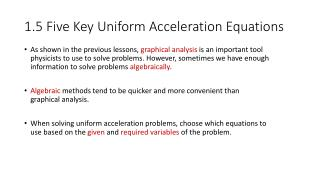# 1.5 Five Key Uniform Acceleration Equations - PowerPoint PPT PresentationDownload Presentation1.5 Five Key Uniform Acceleration Equations

1.5 Five Key Uniform Acceleration EquationsDownload Presentation## 1.5 Five Key Uniform Acceleration Equations

- - - - - - - - - - - - - - - - - - - - - - - - - - - E N D - - - - - - - - - - - - - - - - - - - - - - - - - - -
##### Presentation Transcript

1. 1.5 Five Key Uniform Acceleration Equations • As shown in the previous lessons, graphical analysis is an important tool physicists to use to solve problems. However, sometimes we have enough information to solve problems algebraically. • Algebraic methods tend to be quicker and more convenient than graphical analysis. • When solving uniform acceleration problems, choose which equations to use based on the given and required variables of the problem.

2. 1.5 Five Key Uniform Acceleration Equations • To be able to solve uniform acceleration problems, we need to derive algebraic equations from a change in velocity using a standard graph . • The 1st Equation stems from the area under the graph

3. 1.5 Five Key Uniform Acceleration Equations • The 2nd Equation stems from the slope of the graph and rearranging for .

4. 1.5 Five Key Uniform Acceleration Equations • In order to produce the 3rd Equation, we substitute Equation 2 into Equation 1 for as shown below. • Equations 4 and 5 are similarly produced via substitution, by rearranging Equation 2 for and ∆t.

5. 1.5 Five Key Uniform Acceleration Equations • SP #1-3 p.38-39

6. 1.5 Five Key Uniform Acceleration Equations • It is important to note that these five equations are used only when an object undergoes acceleration, a change in velocity. • If an object is travelling at a constant velocity, we simply use the equation for constant velocity from section 1.2.

7. 1.5 Homework • Practice # 1,2 p.39 • Questions #1-6 p.39